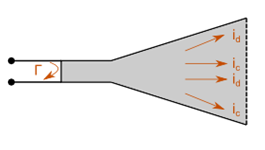#### Antenna efficiency toolReflection, conduction and dielectric losses.

The total antenna efficiency takes into account losses at the input terminals and within the structure of the antenna. The losses may be due, referring to the figure below, to:

• Reflections due to the mismatch between the transmission line and the antenna
• I2R losses (conduction and dielectric)
• This tool calculates the total antenna efficiency using the following equation:

e0= er ecd

where

e0 = total antenna efficiency

er = reflection (mismatch) efficiency = (1 - |Τ|2)